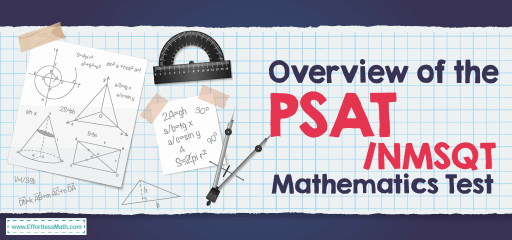# Overview of the PSAT / NMSQT Mathematics TestThe PSAT / NMSQT test or Preliminary SAT/National Merit Scholarship Qualifying Test is a standardized test administered by the College Board and co-sponsored by the National Merit Scholarship Corporation (NMSC) in the United States. Students can practice on the test while simultaneously competing for scholarships based on their performance. There are five parts to this test that take two hours and 10 minutes to complete.

The PSAT mathematics part consists of two sub-sections: a 25-minute no-calculator section, and a 45-minute – calculator-optional section. The no-calculator section tests math fluency and sense of number. This focus is more on the ability to organize and use quantitative information in everyday situations. The calculator section provides an opportunity to demonstrate more complex reasoning skills. Students demonstrate the ability to use procedures and theorems to solve complex equations. However, there are some questions in the calculator section that can be solved faster without using the device.

These sections consist of a total of 48 questions: 40 multiple choice questions and 8 student-produced response (or “grid-in”) questions. Multiple choice questions offer four possible answers. Students have to determine which of the four options is the correct solution to the question. The grid-in questions require students to calculate and grid the correct answers, no answer options are given. Several grid-in questions can have more than one correct answer.

## What does cover in the PSAT math test?

The PSAT mathematic questions focus on four areas:

### 1- Heart of Algebra:

This section assesses the student’s ability to solve fluently and create linear equations and inequalities and their analytical skills. Some of the problems in this area may be simple, while others pose challenges to strategy and understanding. The questions in this section consist of multiple-choice questions and student-produced responses.

Many Hearts of Algebra questions ask students to do one or more of the following: Define at least one variable that represents the quantities in question, Write at least one equation, inequality expression, or function that represents the relationship in question, or solve an equation and interpret the solution in terms of what the question is asking.

### 2- Problem & Data Analysis:

This section of the PSAT math measures the test taker’s ability to understand and use quantitative reasoning about ratios, rates, and proportional relationships. Some questions present information about the relationship between two variables in a scatterplot, graph, or table. This section will also ask you questions about your understanding of essential concepts in statistics as well as assessing your conceptual meaning of standard deviation (not to calculate it).

This section of PSAT math focuses on the ability to work and analyze complex equations. The questions may ask you to demonstrate procedural skills in subtracting, adding, and multiplying polynomials and factoring polynomials. This section of the exam examines topics that are important to students before studying at more complex levels of mathematics.

Some of the things that may be covered in this part of the exam include creating equivalent expressions involving rational exponents, creating a quadratic or exponential function, determining the most appropriate form of an expression, and creating an equivalent form of an algebraic expression.

### 4- Additional Topics in Math:

This section of the PSAT math includes average, median, data interpretation, statistics, and mode; probability.

## Do you get a formula sheet on the PSAT?

You need to know the formulas for both the calculator and the non-calculator sections. Most of them are supposed to be given to you, but you need to know when to use them and how to manipulate them to get the desired result.

## How is the PSAT mathematics test scored?

Your math section scored on a scale of 160 to 760. The closer you get to 760, the stronger your mathematics skills are. If you are currently taking this test as a way to assess your relative skill level, a score of 500 or higher means you have above-average math skills. While these scores do not end at your college application, colleges view PSAT scores before applying to students. Having good grades can get your attention, and there are benefits such as application fee waivers, free meals from the colleges that want you to come to their school.

PSAT Math FREE Resources:

## The Best Books to Ace the PSAT Math Test

### What people say about "Overview of the PSAT / NMSQT Mathematics Test - Effortless Math: We Help Students Learn to LOVE Mathematics"?

No one replied yet.

X
41% OFF

Limited time only!

Save Over 41%

SAVE \$10

It was \$24.99 now it is \$14.99